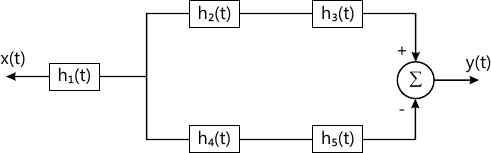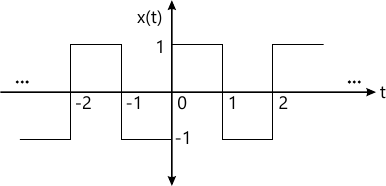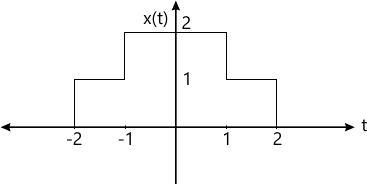MORE IN Signals & Systems
Total marks: --
Total time: --
INSTRUCTIONS
(1) Assume appropriate data and state your reasons
(2) Marks are given to the right of every question
(3) Draw neat diagrams wherever necessary

Solve any one question from Q1 and Q2
1 (a) Check whether the following signals are energy or power. Also find the values of energy or power: $i) \ x(t) = e^{-3t}u (t) \\ ii) \ x[n] = \left ( \dfrac {1}{2} \right )^n u[n]$
6 M
1 (b) Determine the convolution sum of the following sequence using equation of convolution sum. $i) x[n] = \{1, 1, \underset{\uparrow}{2}, 1 \} \\ ii) \ h[n] = u[n] -u[n-3]$
6 M

2 (a) Check whether the following system is:
i) Static/dynamic
ii) Causal/non-causal
iii) Linear/Non-linear
iv) Time invariant/Time variant.
6 M
2 (b) For the system shown in Figure No. 1 determine the overall impulse response if h1(t)=u(t), h2(t)=h5(t)= δ(t), h3(t)=δ(t+2), h4(t)=δ(t-2).6 M

Solve any one question from Q3 and Q4
3 (a) Find trigonometric Fourier series of the signal shown in Figure No. 2.6 M
3 (b) Determine the initial final values of the signal having Laplace transform: $X(s) = \dfrac {2s+3}{s^3 + 2s^2 + 5s}$
6 M

4 (a) Determine the Fourier transform of the following signals.
i) x(t)=e-3|t|6 M
4 (b) Determine transfer function and impulse response of the system represented by: $\dfrac {d^2 y (t)}{dt^2} + 3 \dfrac {dy(t)}{dt} + 2y(t) = 3 \dfrac {dx(t)}{dt} + 2x (t)$ Assume zero initial conditions.
6 M

Solve any one question from Q5 and Q6
5 (a) State and prove any three properties of Energy spectral density (ESD).
6 M
5 (b) Find autocorrelation and power of the signal. x(t)=3 cos ω0t.
7 M

6 (a) Determine the cross correlation of the given signals by graphical method only. $x_1[n] = \{ 1, \underset{\uparrow}{2}, 1\} \\ ii) x_2[n]= \{ 2, \underset{\uparrow}{1}, 2\}$
6 M
6 (b) Determine ESD of the given signal and determine its energy using the relation between ESD and energy: x(t) =e2tu(-t).
7 M

Solve any one question from Q7 and Q8
7 (a) State and explain the properties of cumulative distribution function (CDF).
6 M
7 (b) Determine mean, mean square, variance and standard deviation for the given probability density function (PDF): \begin {align*} f_x(x) &=x^2 \text{ for } &0\le x \le 1 \\ &=0 &\text{ otherwise } \end{align*}
7 M

8 (a) A box contains five white balls, 6 blue balls and three yellow balls. A ball is drawn at random. Find probability that:
i) ball is not yellow
ii) ball is either white or yellow
In the second random experiment if two balls are drawn in succession, then what is the probability that the second ball is blue if the first ball is white.
6 M
8 (b) For the CDF given by, \begin {align*} F_x (x) &=0, &x<0 \\ &=\dfrac {2x}{5}, &0\le x \le 2 \\ &=k, &x>2 \end{align*} Find k, PDF, P(12).
7 M

More question papers from Signals & Systems